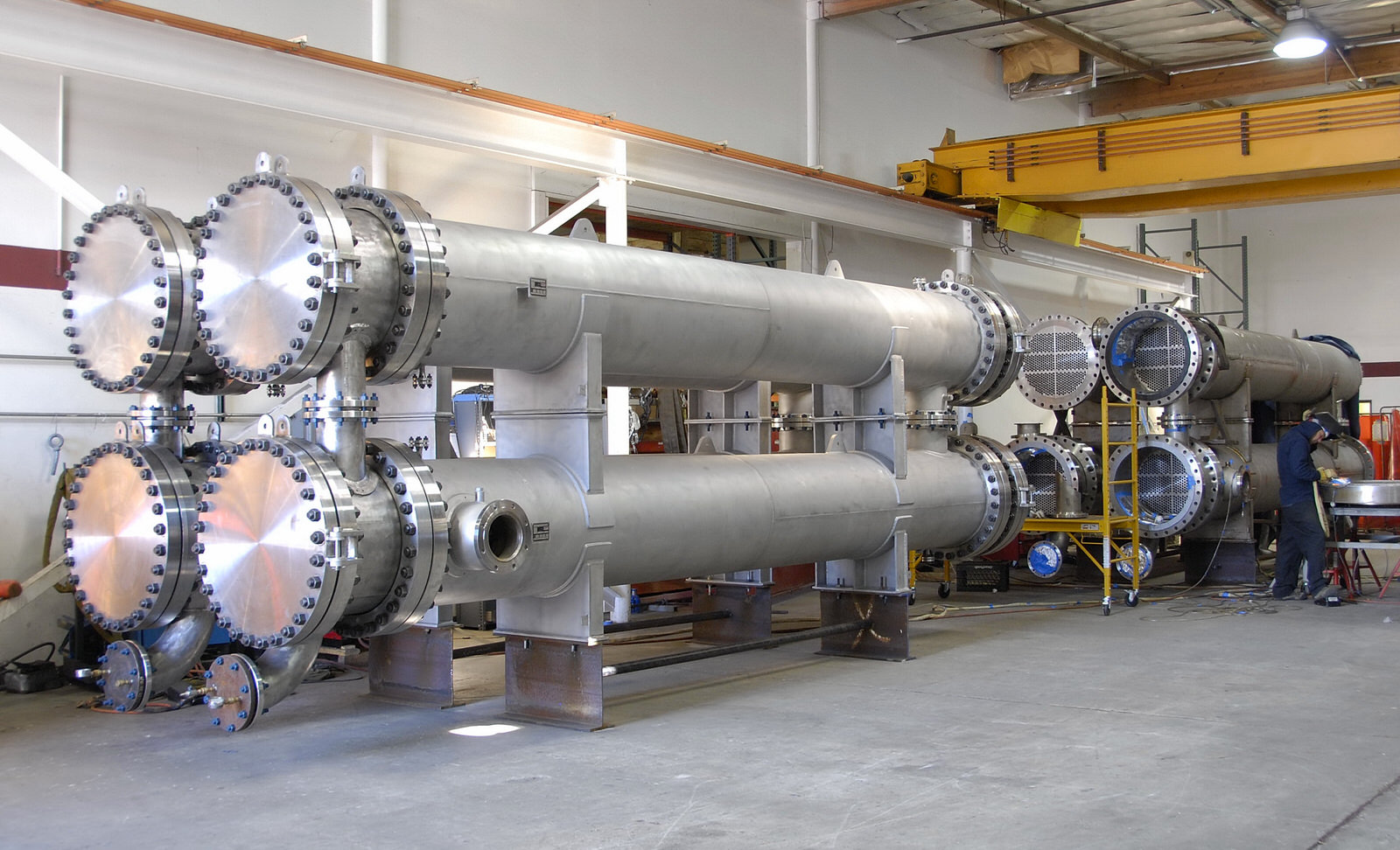Skip to main content# Design of Shell and Tube Heat Exchanger

It’s been quite a long time since the last time I saw and designed shell and tube heat exchanger. Recently, I got opportunity to dig and refresh knowledge that type of heat exchanger. In this post, I want to share you about design of shell and tube heat exchanger.

This figure shows you general step-by-step method of design of shell and tube heat exchanger.Design of shell and tube heat exchanger

This is the explanation:

1. Define the duty (Q) from mass flow rate, inlet temperature, outlet temperature, and heat capacity. I usually calculate for both shell side and tube side just to ensure that the duty is similar. If you have heat capacity properties in inlet side and outlet side, I recommend to use average value. Sometimes, in sizing we have to add contingency, for example 10%. So the calculated duty shall be multiplied by 110%.
2. Collect physical properties. It is very clear step. Use average value for all properties.
3. Select trial value of overall heat transfer coefficient (Uguess). There is particular graph to estimate overall heat transfer coefficient. The graph is a function of process fluid heat transfer coefficient and service fluid heat transfer coefficient.
4. Calculate mean temperature difference (delta Tm). Actually delta Titself is not sufficient. We have to calculate corrected delta Tm which is a function of temperature ratios (R, S) and configuration of shell and tube heat exchanger (number of tube pass, number of shell pass). We will get correction factor (Ft) from nomograph. Corrected is delta Tm x Ft
5. Calculate heat transfer area (A). By using equation A = Q/(U x corrected delta Tm), you will get estimated heat transfer area.
6. Decide exchanger layout. Although this step only contains three words, the actual step is so many. I will explain this step in separate section
7. Calculate individual heat transfer coefficient (shell side and tube side). For shell side, heat transfer coefficient is a function of baffle cuttube arrangementReynold number, and Prandtl number. As for tube side, heat transfer coefficient is a function of L/diReynold number, and Prandtl number.
8. Calculate overall heat transfer coefficient. In addition of shell side and tube side heat transfer coefficient, you will also need fouling coefficient at shell side and tube side and also thermal conductivity of tube material to calculate overall heat transfer coefficient. Sometimes fouling coefficients are defined by user/contractor. If this data is not available, we need to estimate.
9. Check if Uguess in step 3 is the same as Ucalculated in step 8. I think this is the hardest part. In the beginning of design, I think we will get big difference between Uguess and Ucalculated. That’s fine. If that happens, revise Uguess and follow the whole step over. Uguess is usually in form of range. Be careful to check several data that is provided from nomographs (we will use many nomographs in design of shell and tube heat exchanger). If you feel satisfied with the results, for example the difference between Uguess and Ucalculated is less than 10%, then follow next step.
10. Calculate pressure drop at shell side and tube side. Check if calculated pressure drop meets requirement. There are two approach to calculate pressure drop in shell and tube heat exchanger: Kern Method and Bell Method. I usually use those two methods and compare which value is satisfied (I use the highest one).
11. The last step is design optimization. The most optimized one is the one with the smallest area. But you need to pay attention to shell side and tube side velocity and pressure drop.

Reference:

Chemical Engineering Design – RK Sinnot

Feature image from Titan Metal Fabricator